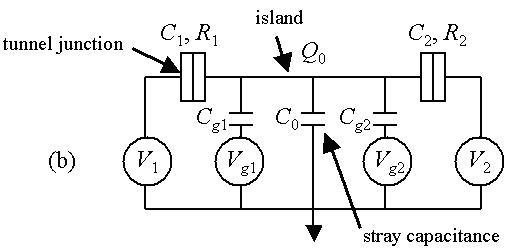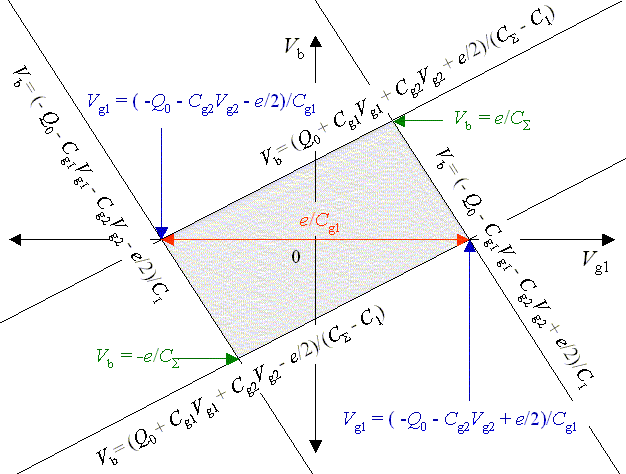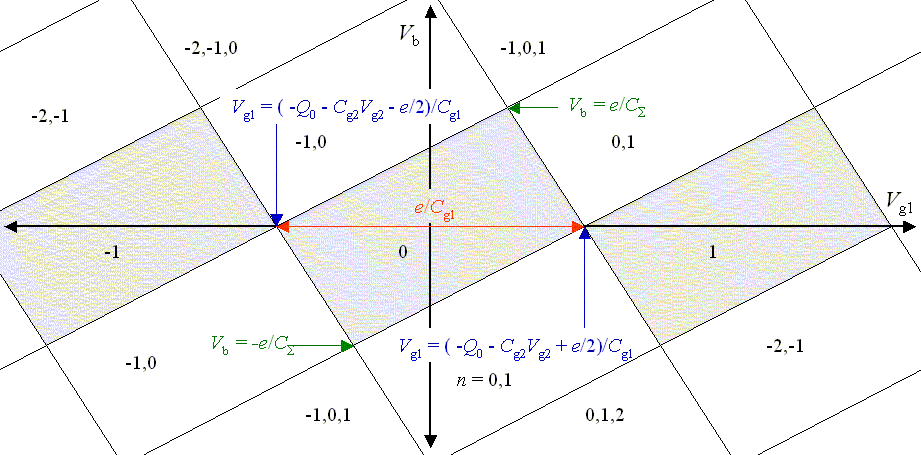## Coulomb blockade in single-electron transistors

This section discusses the electrostatic energy that is required to add or remove an electron from a small conductor and how this leads to a phenomena called the Coulomb blockade. Figure 1(a) shows a small conducting island between source and drain electrodes. These electrodes are close enough that electrons can tunnel to and from the island. Two gates are placed near the island. These two gates are too far away for electrons to tunnel from the island to the gates. Two gates are included in the analysis because two gates are often used. One gate is used to set the bias point and the other is used to input a signal to the SET.Fig. 1 (a) A drawing of a single electron transistor with two gates. (b) The equivalent circuit.

The charge on the island is the sum of the charges on all of the capacitors,

Q = C1(V - V1) + C2(V - V2) + Cg1(V - Vg1) + Cg2(V - Vg2) + C0V.

Here V is the voltage of the island and C0 is the stray capacitance to ground. Ground is assumed to be at zero potential. The other capacitances and voltages in this equation are defined in Fig. 1. The charge on the island can be expressed as the number of electrons on the island plus an offset charge, Q = -ne + Q0. Using this expression, the voltage of the island can be written as,

V(n) = (-ne + Q0 + C1V1 + C2V2 + Cg1Vg1 + Cg2Vg2)/CΣ.

Here CΣ = C1 + C2 + Cg1 + Cg2 + C0 is the total capacitance of the island and e is the positive elementary charge, e = |e|.

The energy it takes to move an infinitesimally small charge dq from ground at zero potential to the island is Vdq. As soon as charge is added to the island, the voltage of the island changes. If q is defined as being the charge that has been added to the island, then the voltage of the island after a charge q has been added is,

V(n) = (-ne + Q0 + q + C1V1 + C2V2 + Cg1Vg1 + Cg2Vg2)/CΣ.

The electrostatic energy necessary to bring a whole electron (charge -e) from ground to the island is,

 -e ∫ V(n)dq = -eV(n) + e²/(2CΣ). 0

Similarly, the electrostatic energy necessary to remove an electron from the island (or equivalently to add a hole of charge e) to ground is,

 e ∫ V(n)dq = eV(n) + e²/(2CΣ). 0

There are four possible single-electron tunnel events in this circuit; an electron can tunnel right through junction 1, it can tunnel left through junction 1, it can tunnel right through junction 2, or it can tunnel left through junction 2. The energy change when an electron tunnels from the left lead onto the island is the same as the energy required to take an electron from the left lead and bring it to ground, eV1, plus the energy to bring an electron from ground to the island, -eV(n) + e²/(2CΣ). Using the expressions given above, the four changes in energies for the four possible single-electron tunneling events are,

ΔE1R(n) = eV1 - e(-ne + Q0 + C1V1 + C2V2 + Cg1Vg1 + Cg2Vg2)/CΣ + e²/(2CΣ),
ΔE1L(n) = -eV1 + e(-ne + Q0 + C1V1 + C2V2 + Cg1Vg1 + Cg2Vg2)/CΣ + e²/(2CΣ),
ΔE2R(n) = -eV2 + e(-ne + Q0 + C1V1 + C2V2 + Cg1Vg1 + Cg2Vg2)/CΣ + e²/(2CΣ),
ΔE2L(n) = eV2 - e(-ne + Q0 + C1V1 + C2V2 + Cg1Vg1 + Cg2Vg2)/CΣ + e²/(2CΣ).

At zero temperature, a tunnel event will only occur if the change in energy is negative. There certain combinations of bias voltages and gate voltages where all four of the changes of energy are positive. In these cases, energy is required to move an electron either way through the tunnel junctions and no tunneling is possible at low temperature. This condition is called the Coulomb blockade. The borders of the Coulomb blockade regions can be found by setting ΔE = 0 in the above equations. Each equation defines a line in the Vb - Vg1 plane. There are two kinds of bias that are commonly encountered in experiments. These are symmetric bias, Vb/2 =V1 = -V2, and asymmetric bias, Vb = V1; V2 = 0. For an asymmetric bias, the four lines that correspond to the charge state n = 0 are plotted in Fig. 2.Fig. 2. The four lines for that define the boundaries of the Coulomb blocakde region are drawn for an asymmetrically biased SET transistor. In the shaded region the change in energy is positive for all four tunnel events and tunneling is forbidden at low temperature. The width of the Coulomb diamond along the Vg1 axis is e/Cg and the diamond extends to ± e/CΣ in bias voltage Vb.

By drawing similar lines for the the other charge states (n = -1, 0, 1, ...) a stability diagram such as the one shown in Fig. 3 can be constructed.Fig. 3. In the gray region labeled 0, tunneling is forbidden by the Coulomb blockade at low temperature and the only stable charge state is the one with zero electrons on the island. The gray region labeled 1 is also a region of Coulomb blockade where the only stable charge state is one electron on the island. When a bias voltage is applied that is great enough to overcome the Coulomb blockade, current flows as electrons tunnel from the source onto the island and then from the island to the drain. In the region labeled 0,1 only one electron at a time can pass through the SET at low temperatures. The charge state keeps changing from zero electrons to one electron as the electrons tunnel on and off the island.